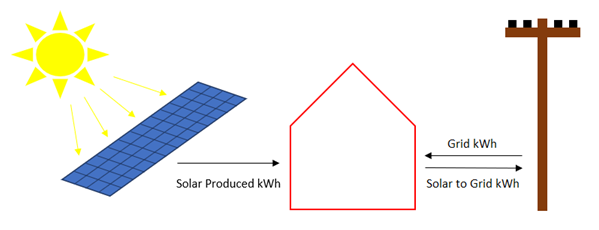# Assumptions and More Definitions Used in the Cases

Let’s first lay out some terms, which will recur in the sections that follow:

## Fit Line kWh vs. Baseline kWh

First, I want to make this point clear.  When we use Metrix or Option C software, we often talk about Baseline kWh.  Baseline represents how much energy “would have been used had there not been an ESP.”

But I want to differentiate between Fit line and Baseline.  The fit line is what you get form the regression of utility data versus CDD, HDD or other variables you may be using.

Sometimes, during the Performance Period, the client may change their building energy usage behavior.  For example, they may start running AHUs all hours or introduce 100% outside air into the air handling units in response to a concern for occupant health.  If they do this in the middle of your Performance Period, then you will see that Actual energy usage will increase and therefore your estimated savings will decrease.  Savings = Baseline – Actual.  To address these changes in building energy usage behavior, ESCOs will add Baseline Modifications to account for this increase (or decrease in usage).  By adding this Baseline Modification, assuming it was estimated correctly, the overall energy savings estimate should remain untainted by the change in energy usage behavior.So the Baseline kWh then becomes:

Baseline kWh = Fit line kWh +/- Baseline Modifications

Where:

Fit line kWh = the kWh that were estimated as Baseline kWh before any baseline modifications were put into place.

Fit line kWh are based on the regression equation of base year bills.

## Building, Solar Produced, Grid, Solar to Grid, and Bill kWh

You need not memorize these terms, but in the Solutions section, you will see them, so you will want to use this table as a reference.

 Term Means Building kWh = kWh used by the building Solar Produced kWh = kWh produced by the solar PV system Grid kWh = kWh supplied by the grid to the building Solar to Grid kWh = kWh supplied by the solar PV system to the grid Bill kWh = kWh supplied by grid to building – kWh supplied by solar PV system to grid = Grid kWh – Solar to Grid kWh = Net kWhIn the case where all solar PV power goes to the grid, and the grid feeds the building, then

Grid kWh = Building kWh.

In the case where the solar PV power goes to the building first, then

Building kWh = Solar Produced + Grid kWh, and

Grid kWh = Bill kWh.